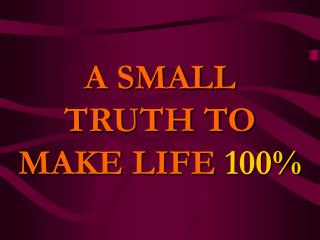# A SMALL TRUTH TO MAKE LIFE 100% - PowerPoint PPT PresentationDownload PresentationA SMALL TRUTH TO MAKE LIFE 100%

A SMALL TRUTH TO MAKE LIFE 100%
Download Presentation## A SMALL TRUTH TO MAKE LIFE 100%

- - - - - - - - - - - - - - - - - - - - - - - - - - - E N D - - - - - - - - - - - - - - - - - - - - - - - - - - -
##### Presentation Transcript

1. A SMALL TRUTH TO MAKE LIFE 100%

2. If A B C D E F G H I J K L M N O P Q R S T U V W X Y Z is equal to 1 2 3 4 5 6 7 8 9 10 11 12 13 14 15 16 17 18 19 20 21 22 23 24 25 26

3. Hard Work H+A+R+D+W+O+R+K 8+1+18+4+23+15+18+11 = 98% Knowledge K+N+O+W+L+E+D+G+E 11+14+15+23+12+5+4+7+5 =96%

4. Love L+O+V+E 12+15+22+5 = 54% Luck L+U+C+K 12+21+3+11 = 47% ( don't most of us think this is the most important ??? )

5. Then what makes100% ? Is it Money? ... NO ! ! ! M+O+N+E+Y 13+15+14+5+25 = 72% Leadership? ... NO ! ! ! L+E+A+D+E+R+S+H+I+P 12+5+1+4+5+18+19+9+16 =89%

6. Every problem has a solution, only if we perhaps change our attitude. To go to the top, to that 100%, what we really need to go further... a bit more...

7. ATTITUDE A+T+T+I+T+U+D+E 1+20+20+9+20+21+4+5 = 100% It is OUR ATTITUDE towards Life and Work that makes OUR Life100%! ! !

8. ATTITUDE IS EVERYTHING Change Your Attitude … And You Change Your Life ! ! !

9. Now that you know the answer… WHAT WILL YOU DO ABOUT IT ? THE LEAST YOU CAN DO IS TO SHARE THIS MESSAGE WITH THOSE YOU CARE